# CostVolumeProfit Analysis Chapter 18 Learning Objective 1 Identify

• Slides: 66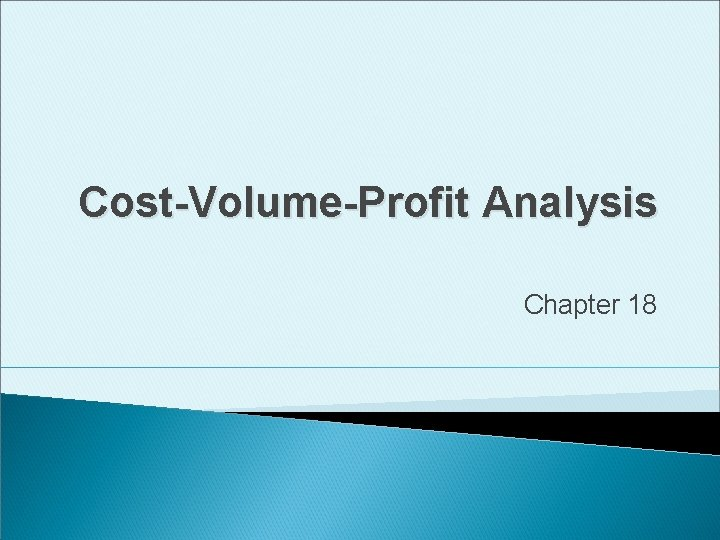Cost-Volume-Profit Analysis Chapter 18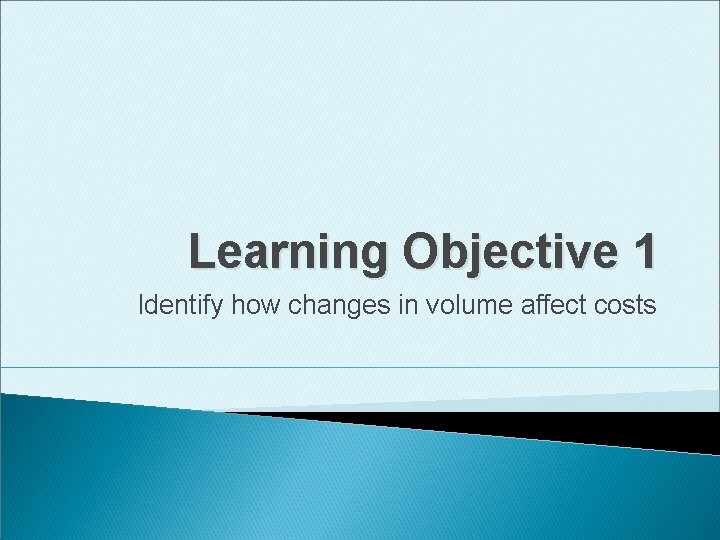Learning Objective 1 Identify how changes in volume affect costs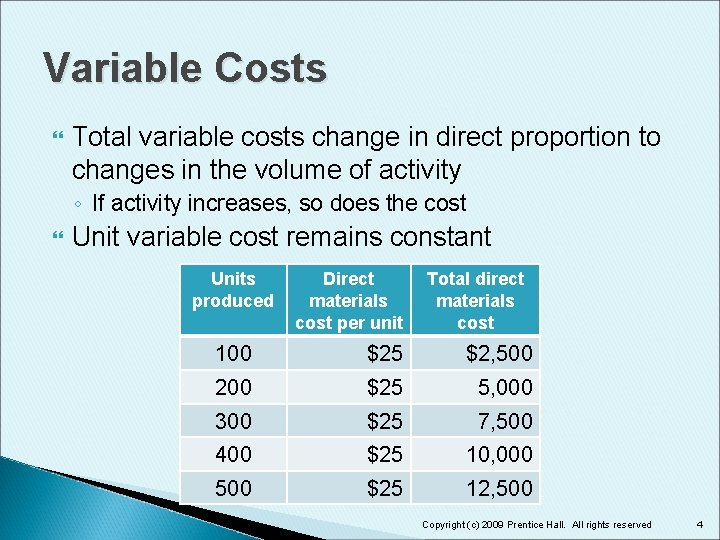Variable Costs Total variable costs change in direct proportion to changes in the volume of activity ◦ If activity increases, so does the cost Unit variable cost remains constant Units produced Direct materials cost per unit Total direct materials cost 100 \$25 \$2, 500 200 \$25 5, 000 300 \$25 7, 500 400 \$25 10, 000 500 \$25 12, 500 Copyright (c) 2009 Prentice Hall. All rights reserved 4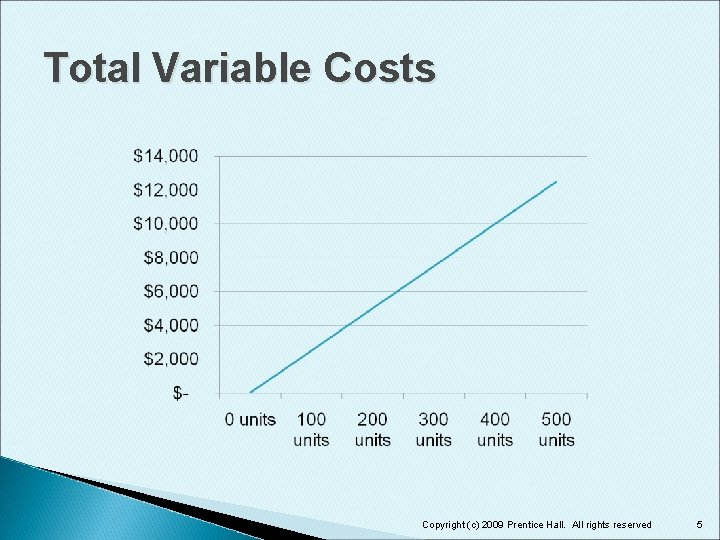Fixed Costs Do not change over wide ranges in volume Examples: ◦ Straight-line depreciation ◦ Salaries for managers Fixed cost per unit is inversely proportional to activity ◦ The more activity, the less the fixed cost per unit Copyright (c) 2009 Prentice Hall. All rights reserved 6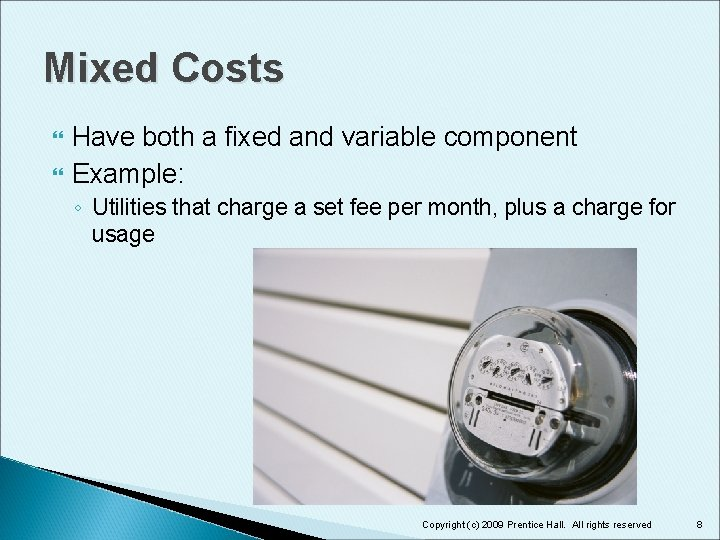Mixed Costs Have both a fixed and variable component Example: ◦ Utilities that charge a set fee per month, plus a charge for usage Copyright (c) 2009 Prentice Hall. All rights reserved 8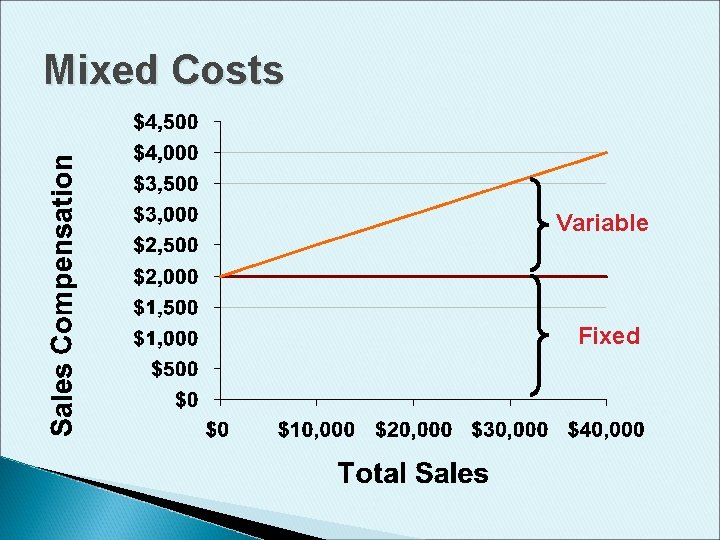Mixed Costs Variable FixedHigh-Low Method to separate mixed costs into variable and fixed components Select the highest level and the lowest level of activity over a period of time Copyright (c) 2009 Prentice Hall. All rights reserved 10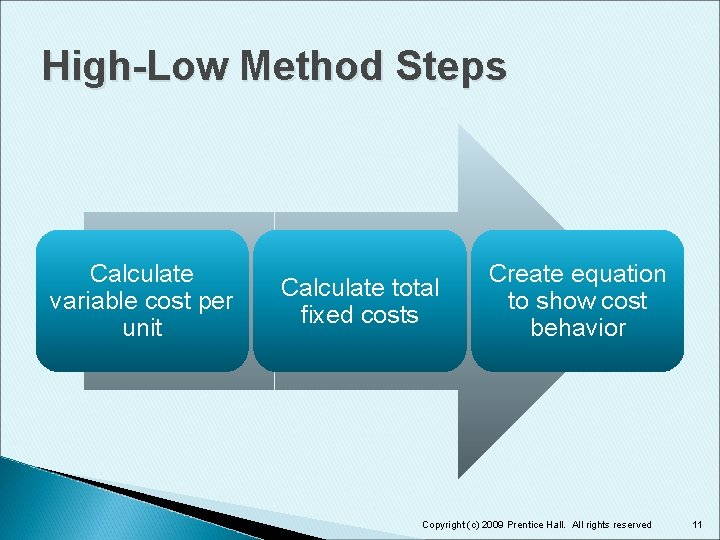High-Low Method Steps Calculate variable cost per unit Calculate total fixed costs Create equation to show cost behavior Copyright (c) 2009 Prentice Hall. All rights reserved 11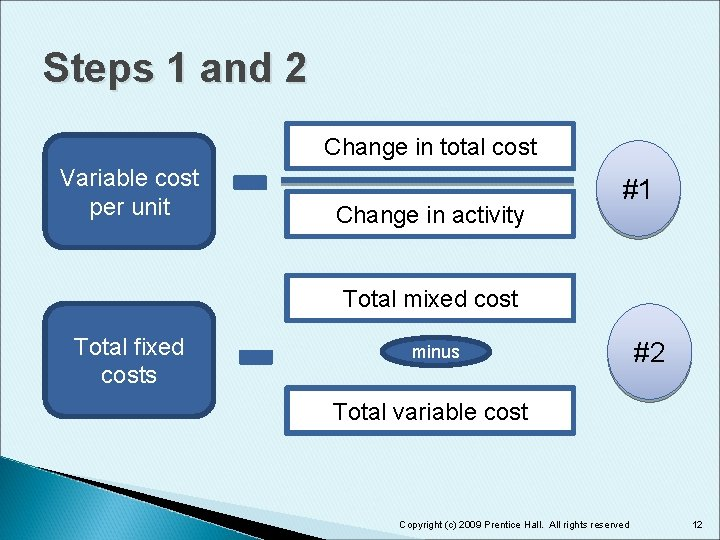Steps 1 and 2 Change in total cost Variable cost per unit Change in activity #1 Total mixed cost Total fixed costs minus #2 Total variable cost Copyright (c) 2009 Prentice Hall. All rights reserved 12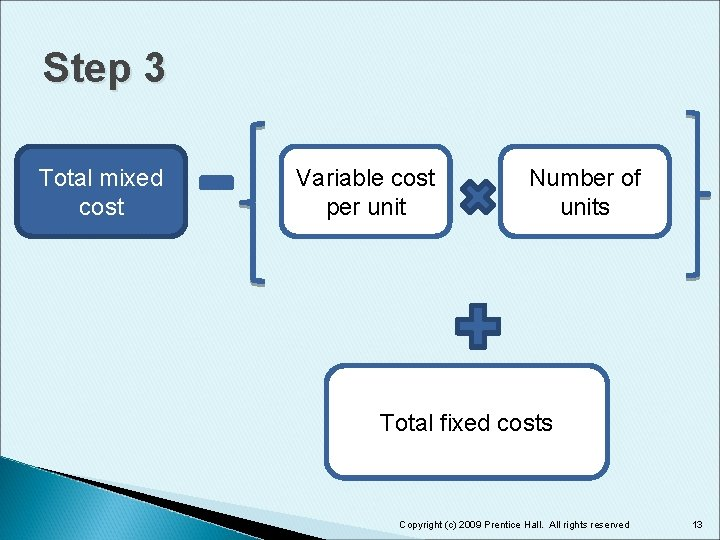Step 3 Total mixed cost Variable cost per unit Number of units Total fixed costs Copyright (c) 2009 Prentice Hall. All rights reserved 13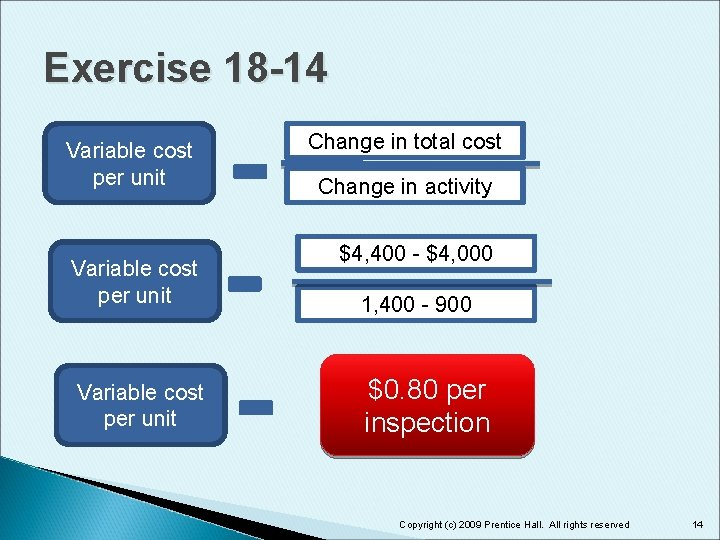Exercise 18 -14 Variable cost per unit Change in total cost Change in activity \$4, 400 - \$4, 000 1, 400 - 900 \$0. 80 per inspection Copyright (c) 2009 Prentice Hall. All rights reserved 14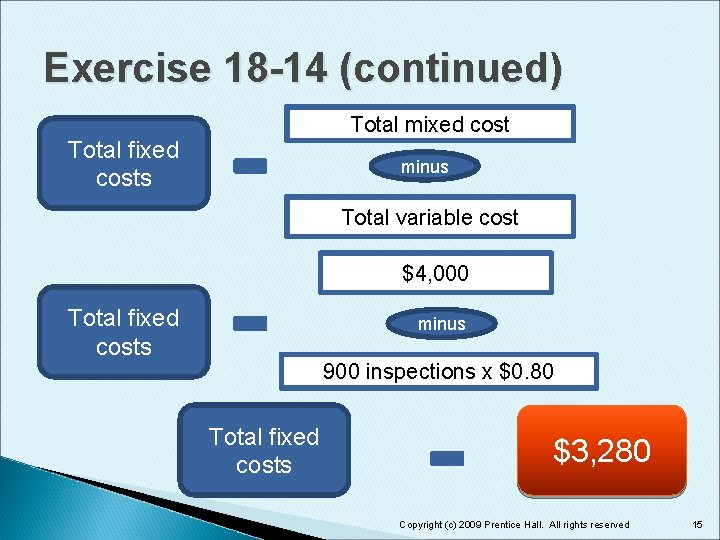Exercise 18 -14 (continued) Total mixed cost Total fixed costs minus Total variable cost \$4, 000 Total fixed costs minus 900 inspections x \$0. 80 Total fixed costs \$3, 280 Copyright (c) 2009 Prentice Hall. All rights reserved 15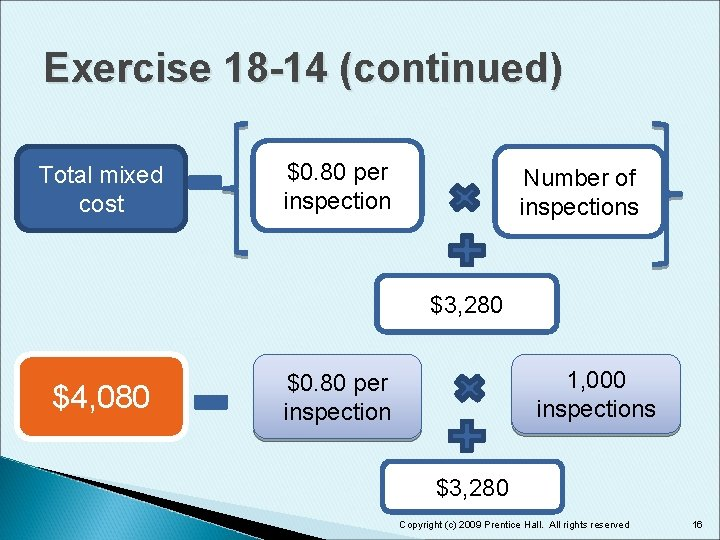Exercise 18 -14 (continued) Total mixed cost \$0. 80 per inspection Number of inspections \$3, 280 \$4, 080 1, 000 inspections \$0. 80 per inspection \$3, 280 Copyright (c) 2009 Prentice Hall. All rights reserved 16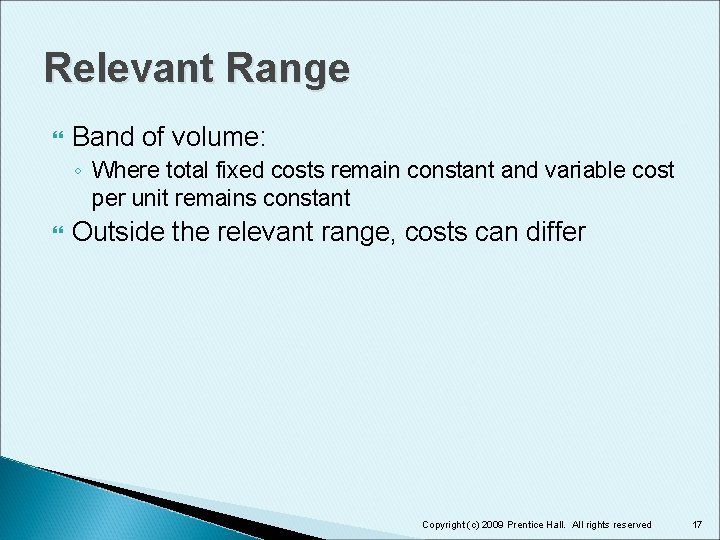Relevant Range Band of volume: ◦ Where total fixed costs remain constant and variable cost per unit remains constant Outside the relevant range, costs can differ Copyright (c) 2009 Prentice Hall. All rights reserved 17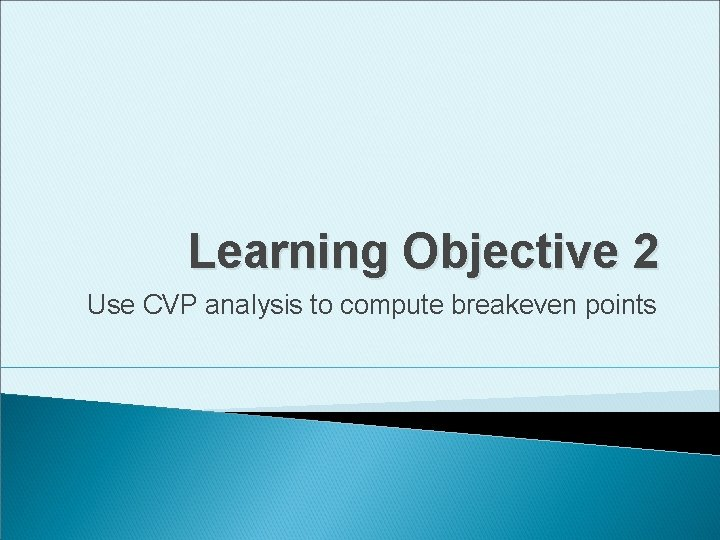Learning Objective 2 Use CVP analysis to compute breakeven points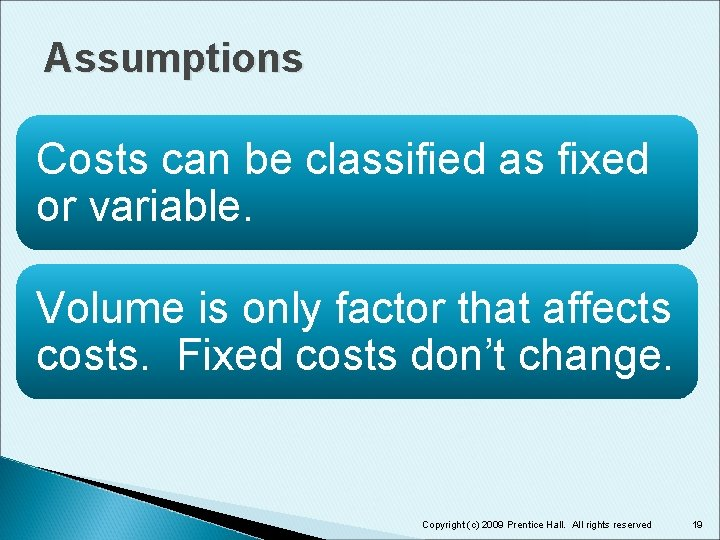Assumptions Costs can be classified as fixed or variable. Volume is only factor that affects costs. Fixed costs don’t change. Copyright (c) 2009 Prentice Hall. All rights reserved 19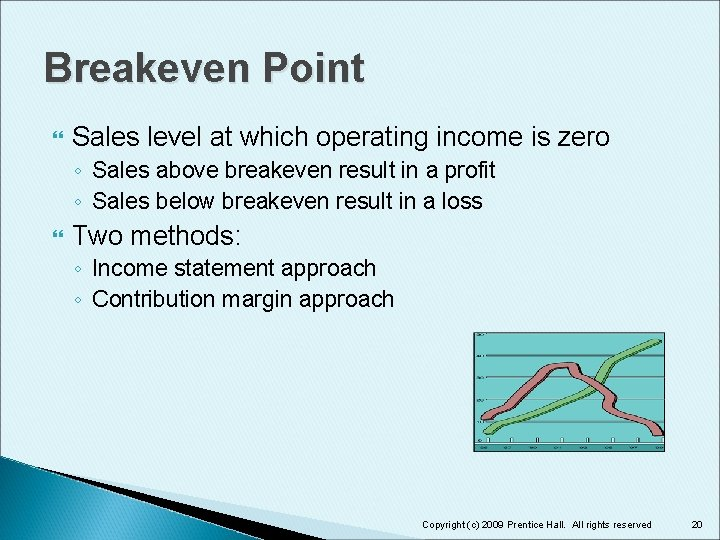Breakeven Point Sales level at which operating income is zero ◦ Sales above breakeven result in a profit ◦ Sales below breakeven result in a loss Two methods: ◦ Income statement approach ◦ Contribution margin approach Copyright (c) 2009 Prentice Hall. All rights reserved 20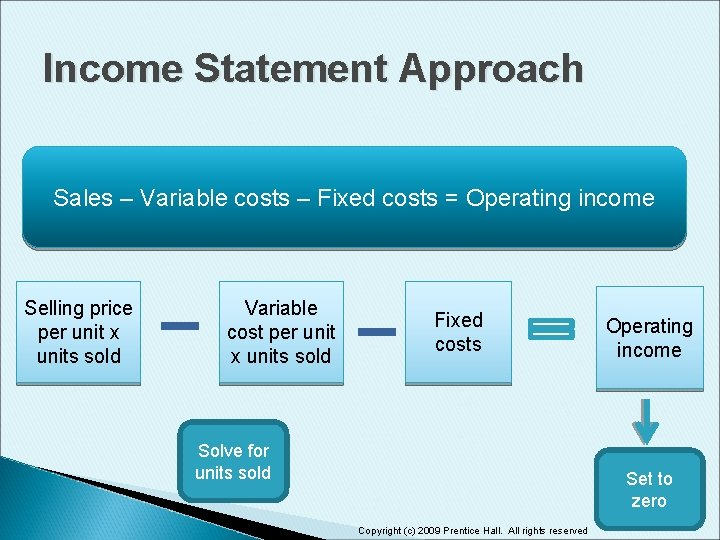Income Statement Approach Sales – Variable costs – Fixed costs = Operating income Selling price per unit x units sold Variable cost per unit x units sold Fixed costs Solve for units sold Operating income Set to zero Copyright (c) 2009 Prentice Hall. All rights reserved 21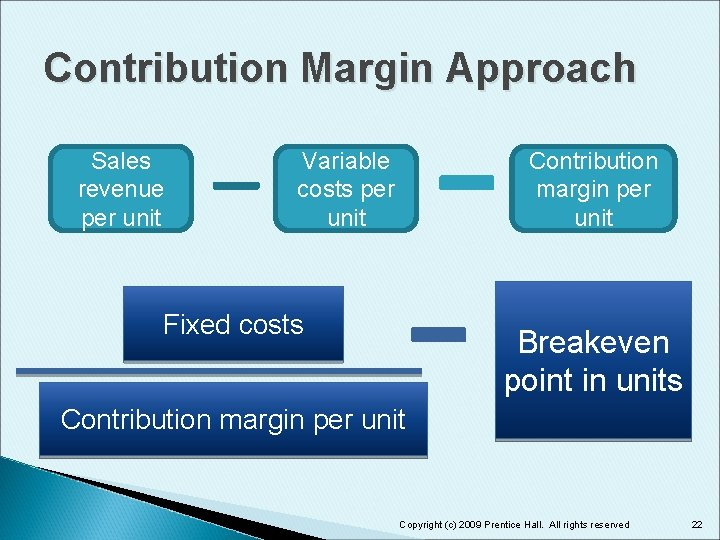Contribution Margin Approach Sales revenue per unit Variable costs per unit Contribution margin per unit Fixed costs Breakeven point in units Contribution margin per unit Copyright (c) 2009 Prentice Hall. All rights reserved 22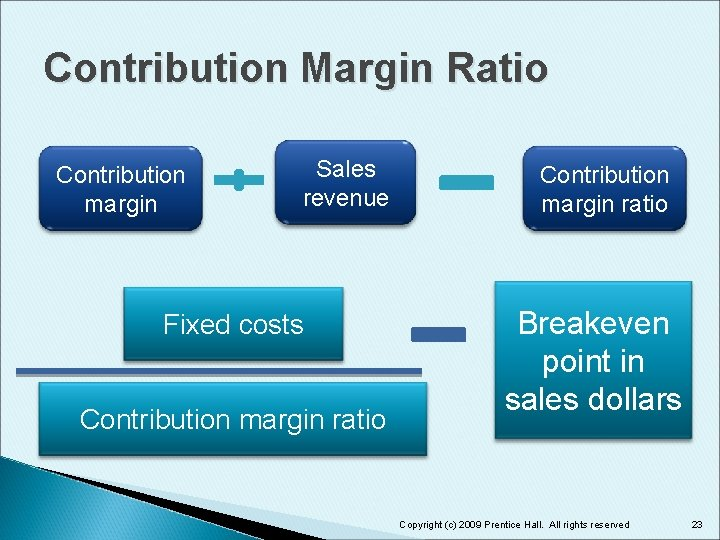Contribution Margin Ratio Contribution margin Sales revenue Fixed costs Contribution margin ratio Breakeven point in sales dollars Copyright (c) 2009 Prentice Hall. All rights reserved 23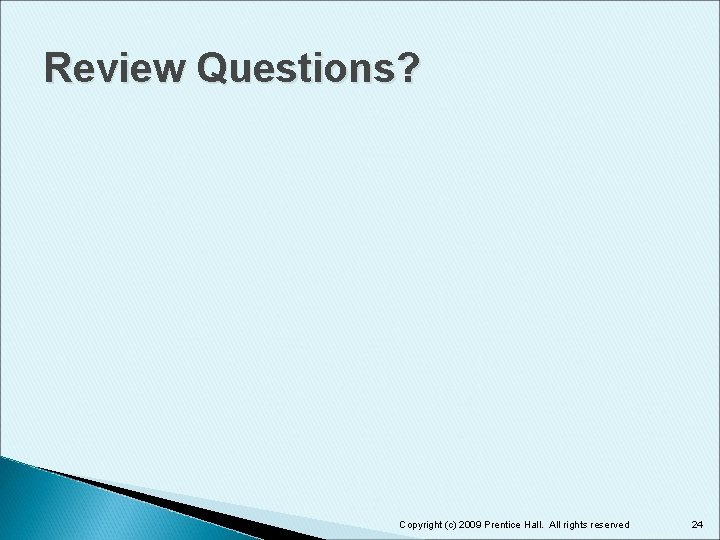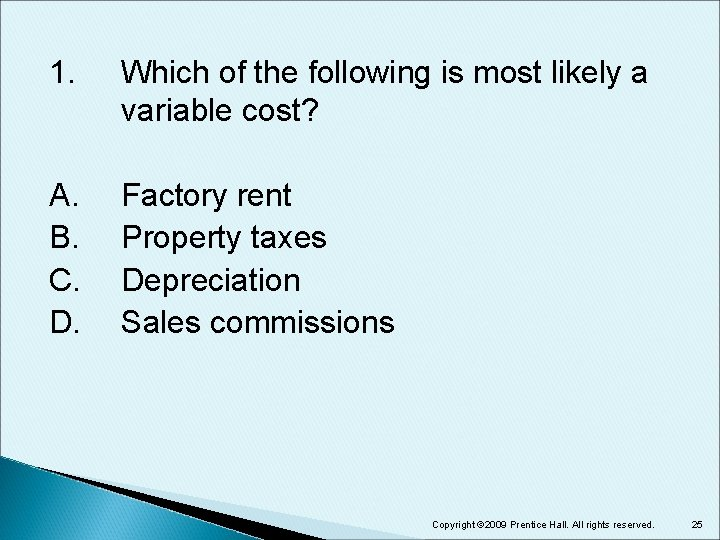1. Which of the following is most likely a variable cost? A. B. C. D. Factory rent Property taxes Depreciation Sales commissions Copyright © 2009 Prentice Hall. All rights reserved. 25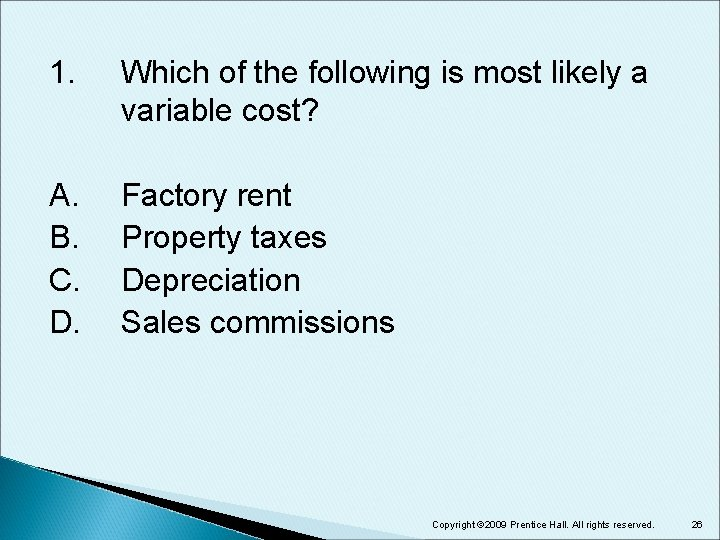1. Which of the following is most likely a variable cost? A. B. C. D. Factory rent Property taxes Depreciation Sales commissions Copyright © 2009 Prentice Hall. All rights reserved. 26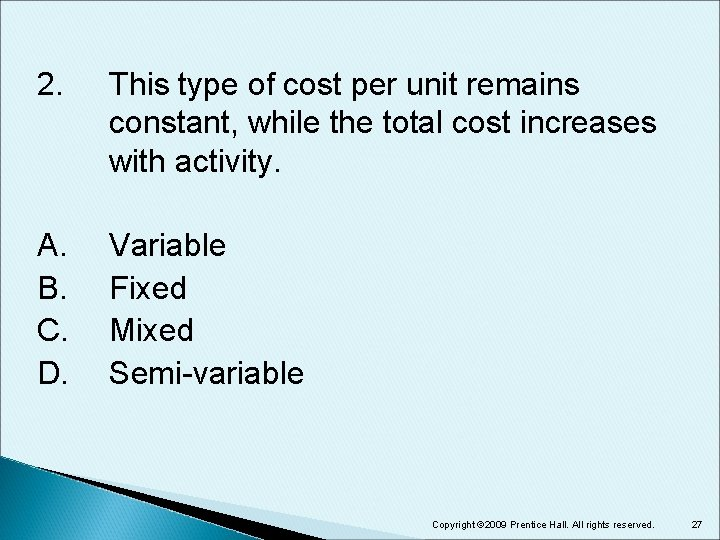2. This type of cost per unit remains constant, while the total cost increases with activity. A. B. C. D. Variable Fixed Mixed Semi-variable Copyright © 2009 Prentice Hall. All rights reserved. 272. This type of cost per unit remains constant, while the total cost increases with activity. A. B. C. D. Variable Fixed Mixed Semi-variable Copyright © 2009 Prentice Hall. All rights reserved. 283. This type of unit cost decreases with activity, but the total cost remains constant. A. B. C. D. Variable Fixed Mixed Semi-variable Copyright © 2009 Prentice Hall. All rights reserved. 293. This type of unit cost decreases with activity, but the total cost remains constant. A. B. C. D. Variable Fixed Mixed Semi-variable Copyright © 2009 Prentice Hall. All rights reserved. 304. Which of the following would most likely be a mixed cost? A. B. C. D. Direct labor Straight-line depreciation Utilities Office salaries Copyright © 2009 Prentice Hall. All rights reserved. 31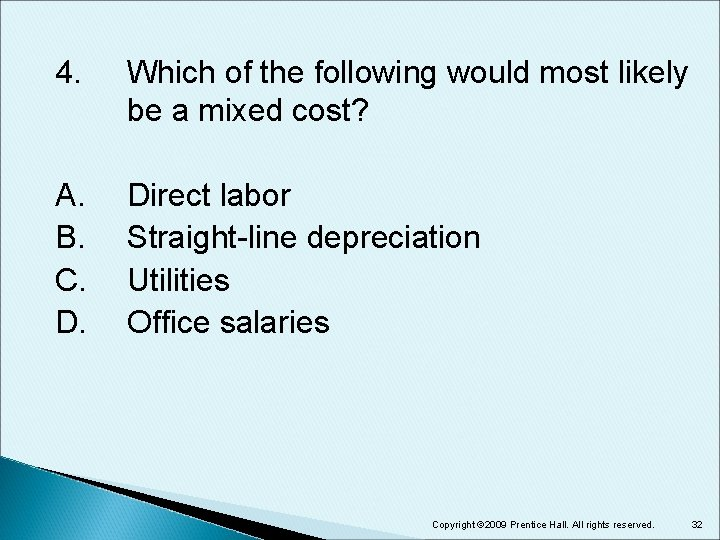4. Which of the following would most likely be a mixed cost? A. B. C. D. Direct labor Straight-line depreciation Utilities Office salaries Copyright © 2009 Prentice Hall. All rights reserved. 32Learning Objective 3 Use CVP analysis for profit planning, and graph the CVP relations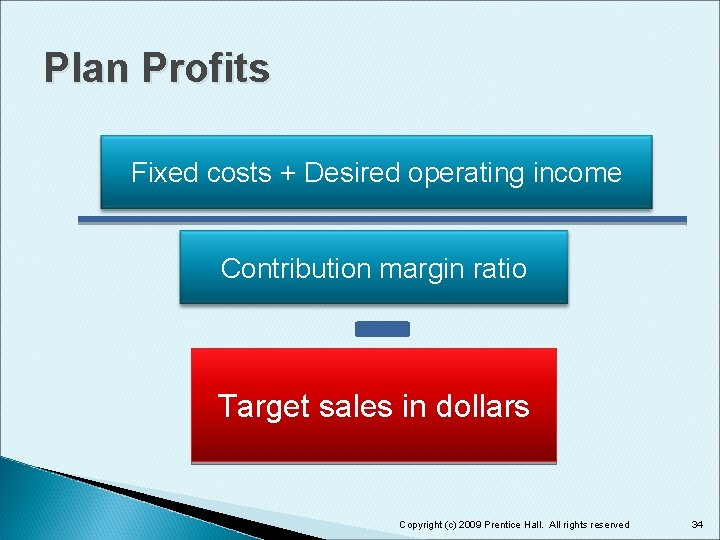Plan Profits Fixed costs + Desired operating income Contribution margin ratio Target sales in dollars Copyright (c) 2009 Prentice Hall. All rights reserved 34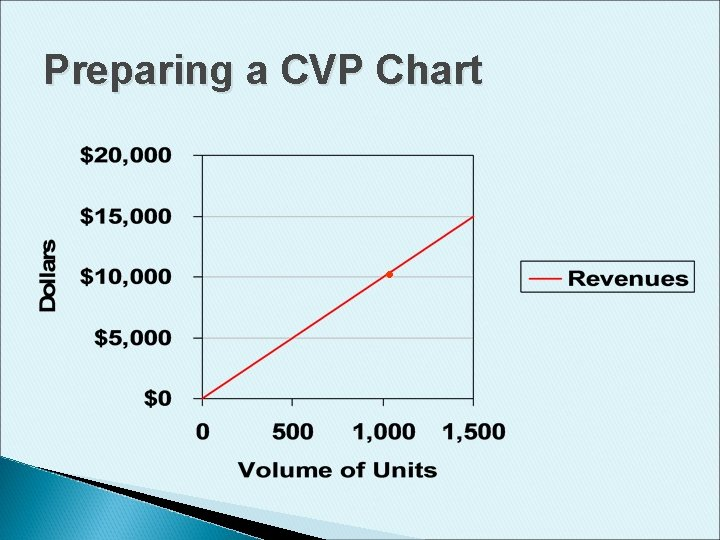Preparing a CVP Chart •Preparing a CVP Chart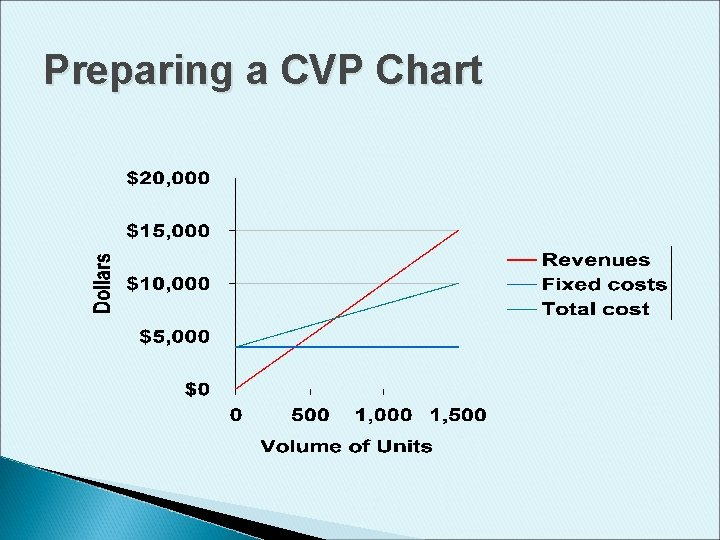Preparing a CVP Chart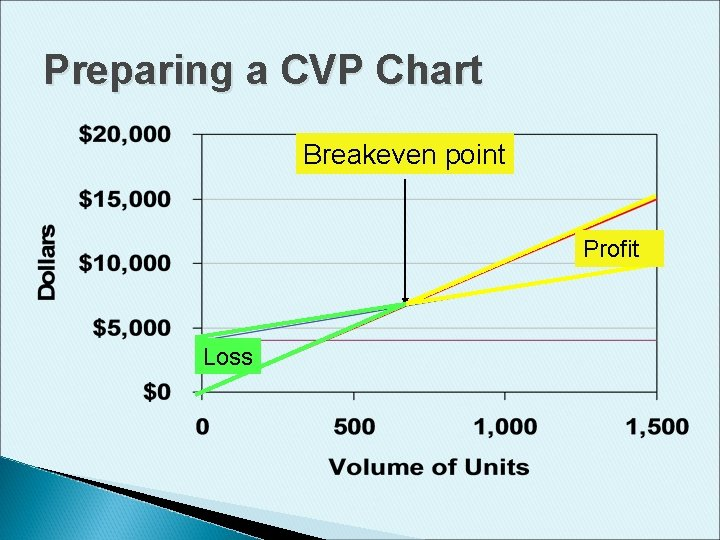Preparing a CVP Chart Breakeven point Profit LossObjective 4 Use CVP methods to perform sensitivity analysis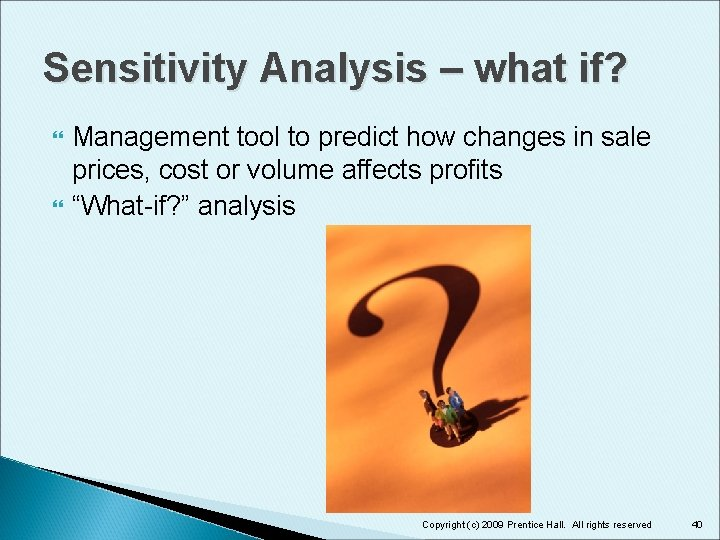Sensitivity Analysis – what if? Management tool to predict how changes in sale prices, cost or volume affects profits “What-if? ” analysis Copyright (c) 2009 Prentice Hall. All rights reserved 40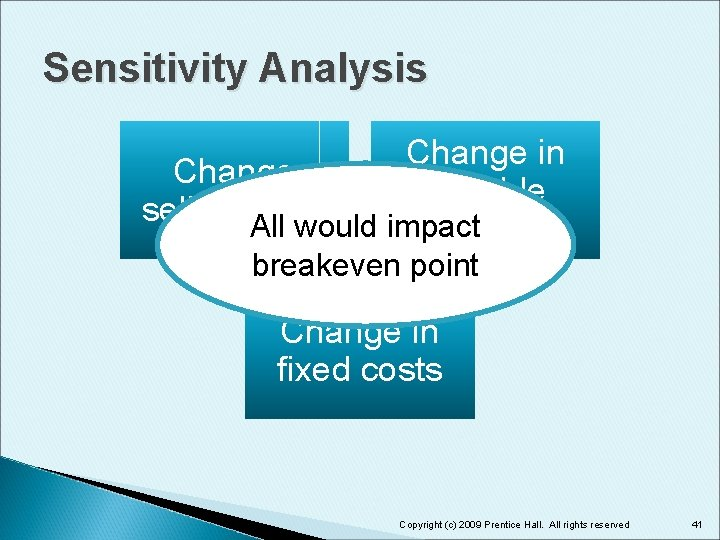Sensitivity Analysis Change in Change variable selling All price would impact costs breakeven point Change in fixed costs Copyright (c) 2009 Prentice Hall. All rights reserved 41Sensitivity Analysis Cause Effect Result Change Contribution margin Breakeven point Selling price increases Increase Decrease Selling price decreases Decrease Increase Variable cost per unit increases Decrease Increase Variable cost per unit decreases Increase Decrease Fixed costs increase No effect Increase Fixed costs decrease No effect Decrease Copyright (c) 2009 Prentice Hall. All rights reserved 42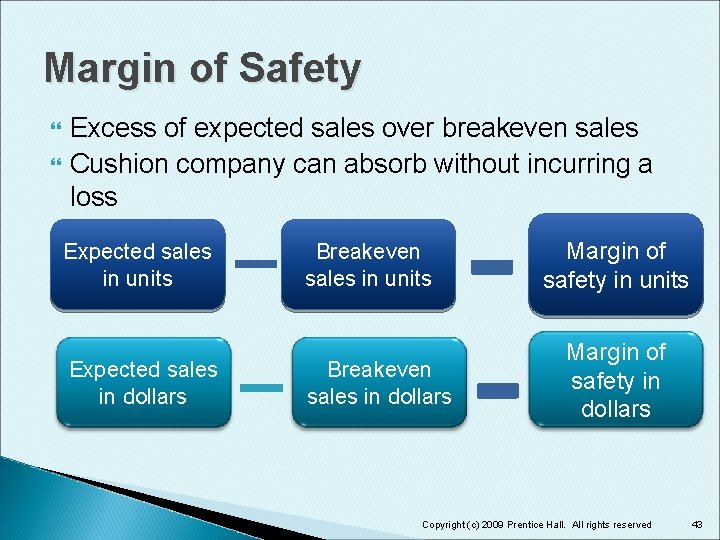Margin of Safety Excess of expected sales over breakeven sales Cushion company can absorb without incurring a loss Expected sales in units Expected sales in dollars Breakeven sales in units Breakeven sales in dollars Margin of safety in units Margin of safety in dollars Copyright (c) 2009 Prentice Hall. All rights reserved 43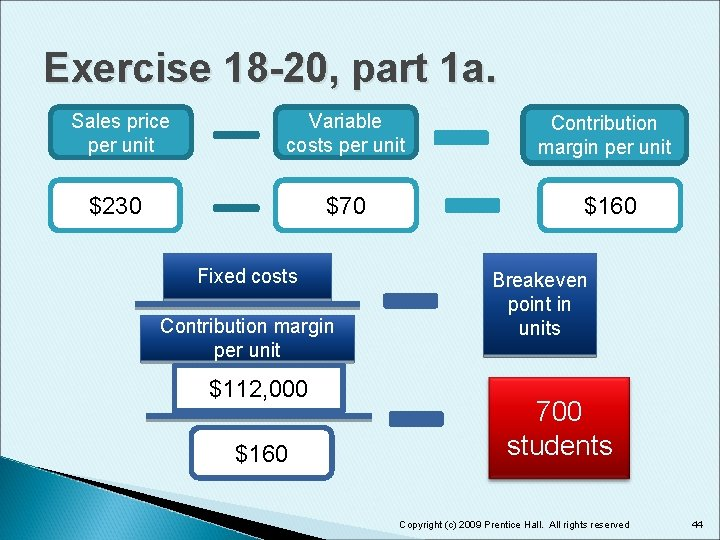Exercise 18 -20, part 1 a. Sales price per unit Variable costs per unit Contribution margin per unit \$230 \$70 \$160 Fixed costs Contribution margin per unit \$112, 000 \$160 Breakeven point in units 700 students Copyright (c) 2009 Prentice Hall. All rights reserved 44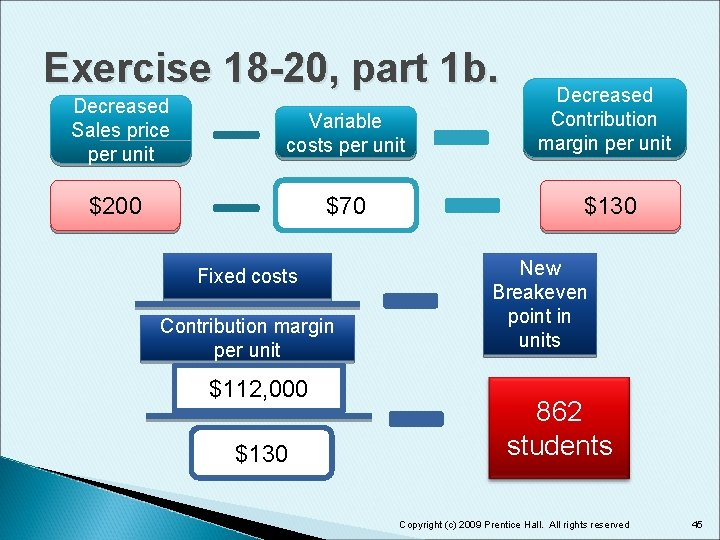Exercise 18 -20, part 1 b. Decreased Sales price per unit Variable costs per unit Decreased Contribution margin per unit \$200 \$70 \$130 Fixed costs Contribution margin per unit \$112, 000 \$130 New Breakeven point in units 862 students Copyright (c) 2009 Prentice Hall. All rights reserved 45Exercise 18 -20, part 1 c. Sales price per unit Decreased variable costs per unit Increased Contribution margin per unit \$230 \$50 \$180 Fixed costs Contribution margin per unit \$112, 000 \$180 New Breakeven point in units 623 students Copyright (c) 2009 Prentice Hall. All rights reserved 46Exercise 18 -20, 1 d. Sales price per unit Variable costs per unit Contribution margin per unit \$230 \$70 \$160 Decreased fixed costs Contribution margin per unit \$102, 000 \$160 Breakeven point in units 638 students Copyright (c) 2009 Prentice Hall. All rights reserved 47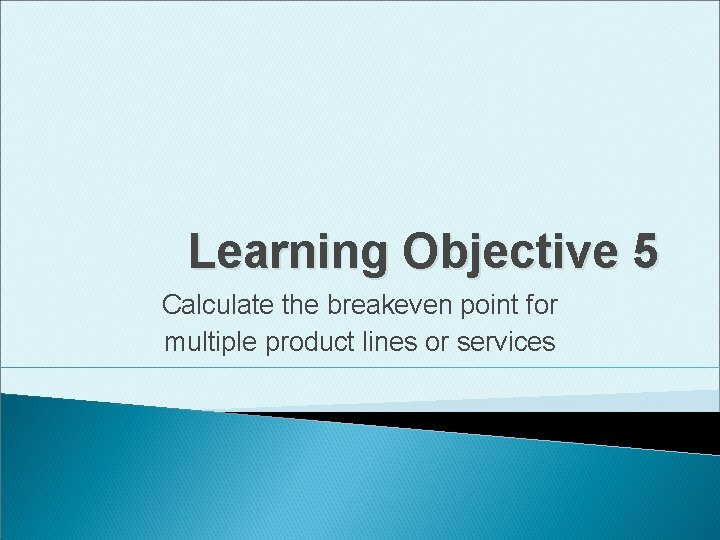Learning Objective 5 Calculate the breakeven point for multiple product lines or servicesMultiple Product Lines Selling prices and variable costs differ for each product ◦ Different contribution to profits Weighted-average contribution margin computed Sales mix provides weights ◦ Combination of products that make up total sales Copyright (c) 2009 Prentice Hall. All rights reserved 49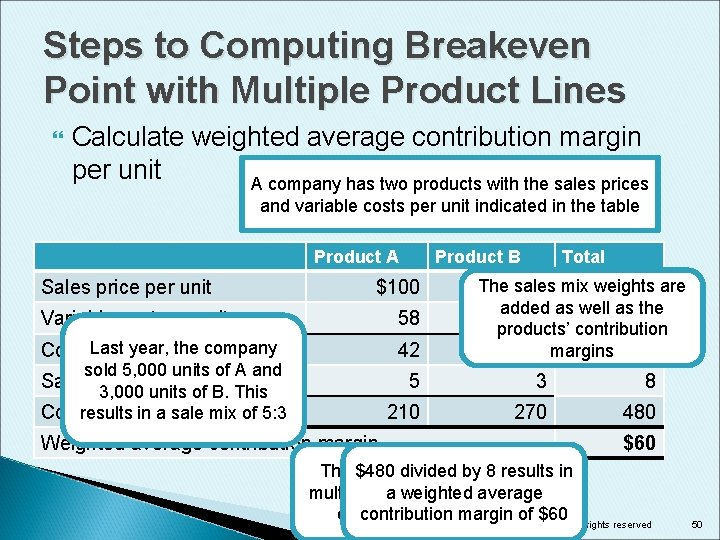Steps to Computing Breakeven Point with Multiple Product Lines Calculate weighted average contribution margin per unit A company has two products with the sales prices and variable costs per unit indicated in the table Product A Sales price per unit Product B \$100 Variable cost per unit 58 Last year, the company Contribution margin per unit 42 sold 5, 000 units of A and Sales mix per unit 3, 000 units of B. This Contribution results in margin a sale mix of 5: 3 Weighted average contribution margin Total The\$150 sales mix weights are added as well as the 60 products’ contribution 90 margins 5 3 8 210 270 480 \$60 The \$480 sales divided mix weight by 8 isresults in multipliedabyweighted the product’s average contribution margin of \$60 Copyright (c) 2009 Prentice Hall. All rights reserved 50Steps to Computing Breakeven Point with Multiple Product Lines Calculate breakeven point for the package of products Fixed costs Weighted average contribution margin per unit assumed \$600, 000 \$60 10, 000 units Copyright (c) 2009 Prentice Hall. All rights reserved 51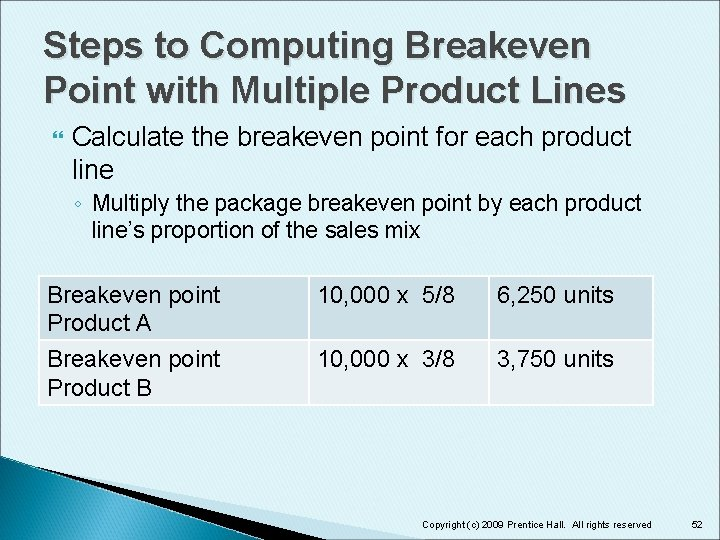Steps to Computing Breakeven Point with Multiple Product Lines Calculate the breakeven point for each product line ◦ Multiply the package breakeven point by each product line’s proportion of the sales mix Breakeven point Product A 10, 000 x 5/8 6, 250 units Breakeven point Product B 10, 000 x 3/8 3, 750 units Copyright (c) 2009 Prentice Hall. All rights reserved 52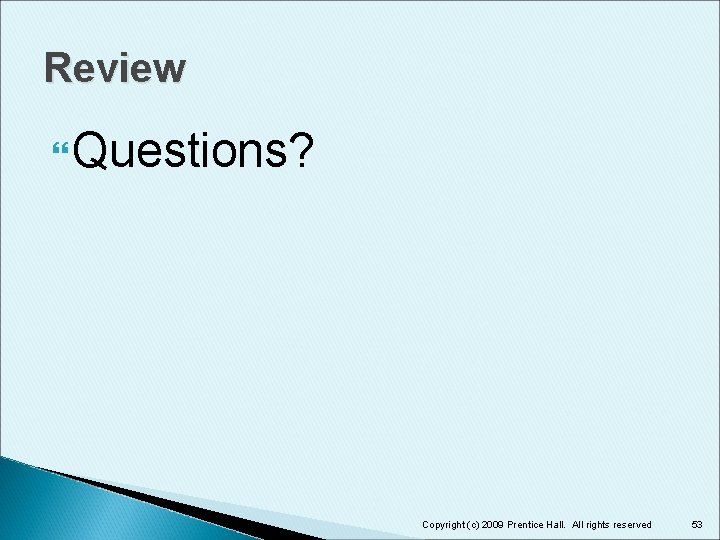5. The simplest method to split a mixed cost into its fixed and variable components is called: A. B. C. D. fixed-variable separation. high-low method. multiple regression. breakeven analysis. Copyright © 2009 Prentice Hall. All rights reserved. 54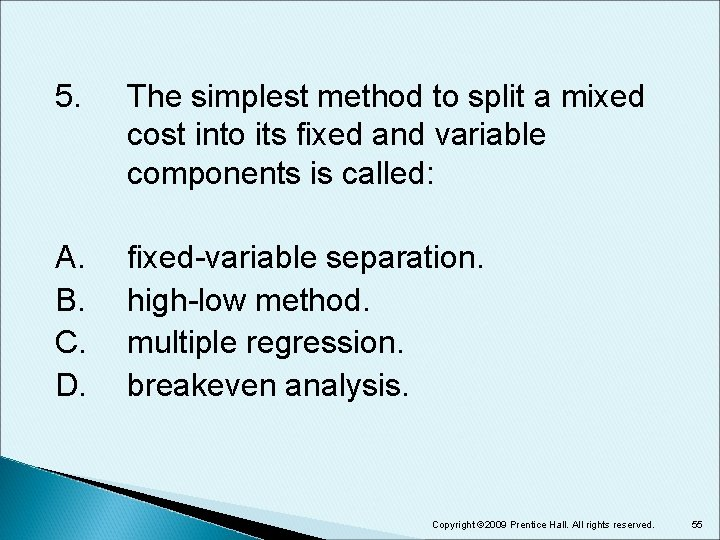5. The simplest method to split a mixed cost into its fixed and variable components is called: A. B. C. D. fixed-variable separation. high-low method. multiple regression. breakeven analysis. Copyright © 2009 Prentice Hall. All rights reserved. 556. Which of the following is an assumption of CVP analysis? A. Costs can be classified as either fixed or variable. Volume is the only factor that impacts costs. Fixed costs don’t change. All of the above are true. B. C. D. Copyright © 2009 Prentice Hall. All rights reserved. 566. Which of the following is an assumption of CVP analysis? A. Costs can be classified as either fixed or variable. Volume is the only factor that impacts costs. Fixed costs don’t change. All of the above are true. B. C. D. Copyright © 2009 Prentice Hall. All rights reserved. 577. The sales level where net income equals zero is called: A. B. C. D. the breakeven point. zero sum sales. net loss. deficit earnings. Copyright © 2009 Prentice Hall. All rights reserved. 587. The sales level where net income equals zero is called: A. B. C. D. the breakeven point. zero sum sales. net loss. deficit earnings. Copyright © 2009 Prentice Hall. All rights reserved. 59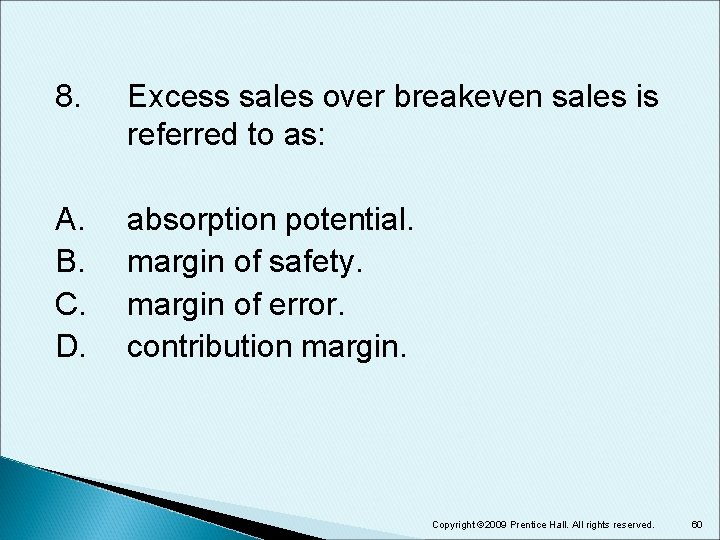8. Excess sales over breakeven sales is referred to as: A. B. C. D. absorption potential. margin of safety. margin of error. contribution margin. Copyright © 2009 Prentice Hall. All rights reserved. 608. Excess sales over breakeven sales is referred to as: A. B. C. D. absorption potential. margin of safety. margin of error. contribution margin. Copyright © 2009 Prentice Hall. All rights reserved. 61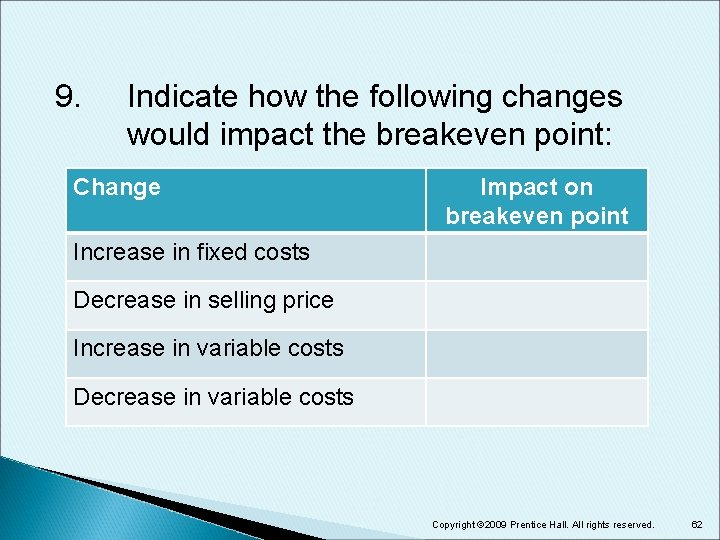9. Indicate how the following changes would impact the breakeven point: Change Impact on breakeven point Increase in fixed costs Decrease in selling price Increase in variable costs Decrease in variable costs Copyright © 2009 Prentice Hall. All rights reserved. 629. Indicate how the following changes would impact the breakeven point: Change Impact on breakeven point Increase in fixed costs Increase Decrease in selling price Increase in variable costs Increase Decrease in variable costs Decrease Copyright © 2009 Prentice Hall. All rights reserved. 6310. Contribution margin equals: A. B. C. D. sales – variable costs. sales – fixed costs – variable costs. sales – variable costs – fixed costs. Copyright © 2009 Prentice Hall. All rights reserved. 64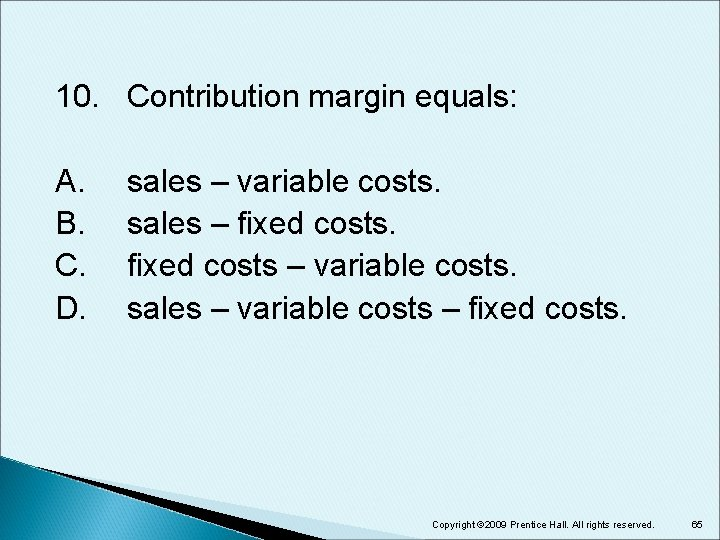10. Contribution margin equals: A. B. C. D. sales – variable costs. sales – fixed costs – variable costs. sales – variable costs – fixed costs. Copyright © 2009 Prentice Hall. All rights reserved. 65End of Chapter 18